+
Shape of a Sampling Distribution
Central Tendency, Variation, and Distributions
0
of 90 possible points

# Shape of a Sampling Distribution

Author: Sophia Tutorial
##### Description:

Identify characteristics of the shape of a sampling distribution using the Central Limit Theorem.

(more)

Sophia’s self-paced online courses are a great way to save time and money as you earn credits eligible for transfer to many different colleges and universities.*

No credit card required

46 Sophia partners guarantee credit transfer.

299 Institutions have accepted or given pre-approval for credit transfer.

* The American Council on Education's College Credit Recommendation Service (ACE Credit®) has evaluated and recommended college credit for 33 of Sophia’s online courses. Many different colleges and universities consider ACE CREDIT recommendations in determining the applicability to their course and degree programs.

Tutorial
what's covered
This tutorial will cover the shape of a sampling distribution. Our discussion breaks down as follows:

1. The Characteristics of Distributions
2. Shape
3. The Central Limit Theorem

## 1. The Characteristics of Distributions

In a sampling distribution, the center is the same as the center of the original distribution. That is to say, the mean of all the x-bar averages is the same as the mean of the original distribution. The shape is also a characteristic of distributions and will be discussed in the next section.

The spread, or standard deviation, is the same as the original standard deviation divided by the square root of sample size. In other words, the standard deviation of all the x-bars is equal to the original standard deviation divided by the square root of n (n being the sample size).

These two characteristics are notated like this:

formula
Mean of a Distribution of Sample Means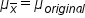Standard Deviation of a Distribution of Sample Means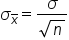## 2. Shape

Consider this spinner: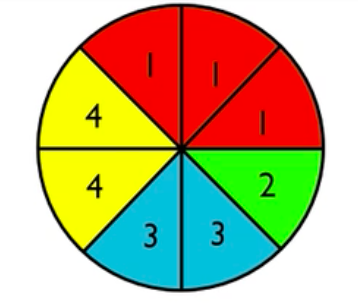Consider the sampling distributions caused by averaging different numbers of spins. Well, it's fairly obvious that if you spun it once, you would spin a one about 3 out of 8 times. You'd spin a two about 1 out of 8 times, a three about 2 out of 8 times, and a four about 2 out of 8 times, making the distribution look something like this: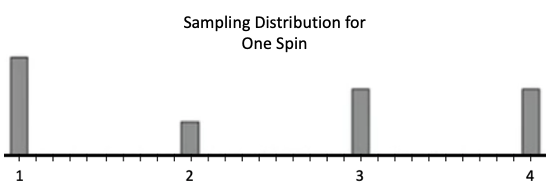You can see here that one is the most common, three and four are the next most common (and equally common), and two is the least common.

What about the sampling distributions if you averaged four spins? Well, you wouldn't just have options of 1, 2, 3, and 4 anymore. You'd have options of 1.25, 1.5, 1.75 , 2, 2.25, 2.5, etc. Having more options would necessarily decrease the likelihood of getting all 1's or all 4's. The distribution would look something like this: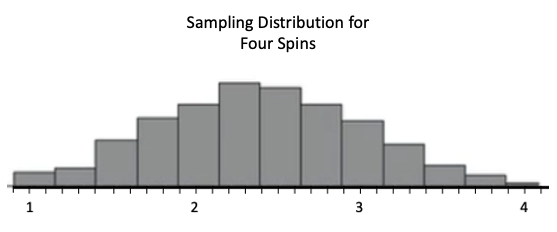Getting all four 1's would be extremely unlikely, and getting all four 4's would also be extremely unlikely. The most likely scenario is getting 2.25. There are a few more 1's than there are anything else, which pulls the mean down a little bit from where you might have thought it would be: 2.3. This distribution is slightly skewed to the right.

What if you sampled nine and averaged nine spins? Well, the probability, for instance, that you get all 1's, therefore averaging a 1, goes down even further. Also, the probability that you get all 4's goes down to almost zero: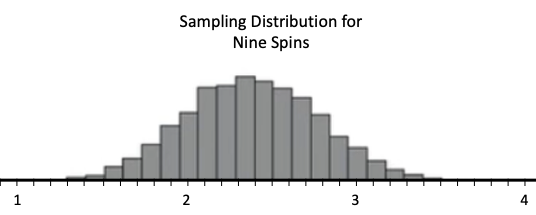As this graph shows, it's possible to get all 1's, but it’s not very likely. It's a lot more common to get something between 2 and 3. The spread of the sampling distribution is decreasing as n gets bigger. As the previous graphs show, the shape of the distribution changes as the number of spins, n, changes.

Suppose you are averaging 20 spins. It's almost impossible to average a 1, a 4, or even something close to 3. You're almost guaranteed to average something between 2 and 3. The spread, again, is decreasing.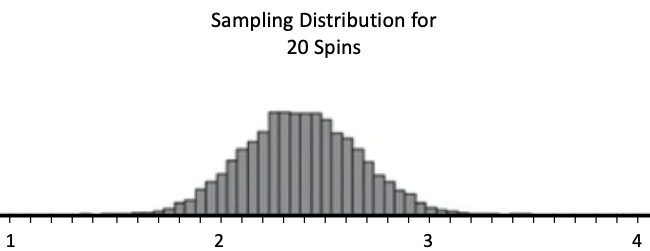What would you say is happening to the shape?

As n increases, the shape is becoming more normal.

## 3. The Central Limit Theorem

The Central Limit Theorem deals with the changes in shape that we saw above; it discusses the shape of a sampling distribution. The Central Limit Theorem states that when the sample size is large, the shape of the sampling distribution of means becomes nearly normal.

brainstorm
How large a sample size is considered large enough for the distribution to be approximately normal? In our distributions so far in this tutorial, is it approximately normal when n is nine? Is it approximately normal when n is 20? What constitutes a large enough sample so that when you show the distribution of all the averages, you get a normal distribution?

The definition of “large” will be different depending on what the original distribution looked like. Our original distribution was almost uniform, so it didn't take very many trials. If the distribution had been heavily skewed, it would have taken more trials to average out some of those high numbers with some of those low numbers.

In most cases, 30 is going to be a good sample size such that when you average the 30 observations, you are going to get something close to what you expect. With a sample size of 30, the distributions that are off from what you expect will tail off in a normal shape.

big idea
For almost all distributions, a sample size of 30 is exactly what we want. It's the Central Limit Theorem that explains why so many real-world processes are normally distributed.

term to know

Central Limit Theorem
A theorem that explains the shape of a sampling distribution of sample means. It states that if the sample size is large (generally n ≥ 30), and the standard deviation of the population is finite, then the distribution of sample means will be approximately normal.

summary
A sampling distribution is the distribution of all possible means of a given size; there are characteristics of distributions that are important, and for the Central Limit Theorem, the important characteristic is the shape. The Central Limit Theorem outlines that when the sample size is large, for most distributions (meaning 30 or larger), then the distribution of sample means will be approximately normal. Occasionally, you need to make the distribution of sample means even bigger than 30 if the parent distribution that you started with is very skewed or has outliers. The Central Limit Theorem is probably the most important idea in statistics.

Good luck!

Source: Adapted from Sophia tutorial by Jonathan Osters.

Terms to Know
Central Limit Theorem

A theorem that explains the shape of a sampling distribution of sample means. It states that if the sample size is large (generally n ≥ 30), and the standard deviation of the population is finite, then the distribution of sample means will be approximately normal.

Formulas to Know
Mean of a Distribution of Sample MeansStandard Deviation of a Distribution of Sample MeansRating